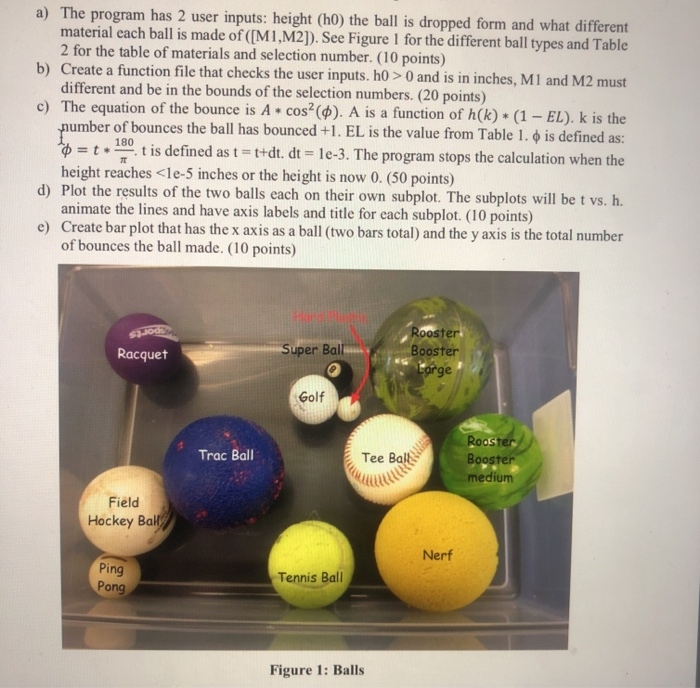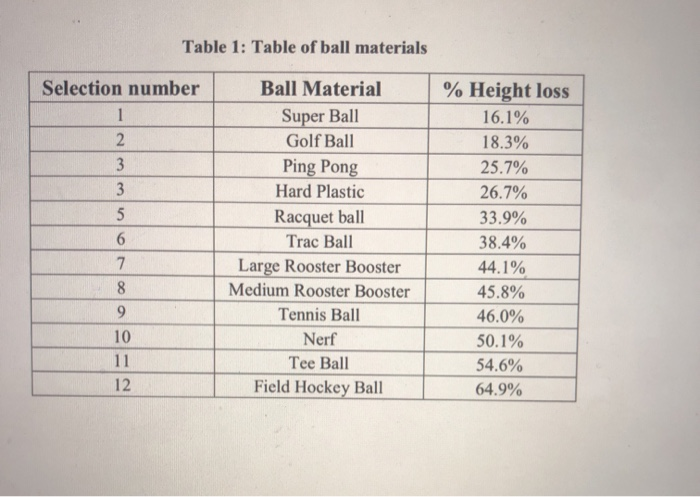# (Solved) : Use Matlab Answer Script Language Used Answer Quesion Matlab Q42699836 . . .thescript language used to answer the quesion is matlaba) The program has 2 user inputs: height (ho) the ball is dropped form and what different material each ball is made of ([M1, M2]). See Figure 1 for the different ball types and Table 2 for the table of materials and selection number. (10 points) b) Create a function file that checks the user inputs. h0 >0 and is in inches, M1 and M2 must different and be in the bounds of the selection numbers. (20 points) c) The equation of the bounce is A * cos(\$). A is a function of h(k) + (1 – EL). K is the number of bounces the ball has bounced +1. EL is the value from Table 1.0 is defined as: = t .t is defined as t = t+dt. dt = 1e-3. The program stops the calculation when the height reaches <le-5 inches or the height is now 0. (50 points) d) Plot the results of the two balls each on their own subplot. The subplots will be t vs. h. animate the lines and have axis labels and title for each subplot. (10 points) e) Create bar plot that has the x axis as a ball (two bars total) and the y axis is the total number of bounces the ball made. (10 points) SIJO Racquet Super Ball Rooster Booster Large Golf Trac Ball Tee Ball Rooster Booster medium Field Hockey Ball Nerf Ping Pong Tennis Ball Figure 1: Balls Table 1: Table of ball materials Selection number 3 6 Ball Material Super Ball Golf Ball Ping Pong Hard Plastic Racquet ball Trac Ball Large Rooster Booster Medium Rooster Booster Tennis Ball | Nerf Tee Ball Field Hockey Ball % Height loss 16.1% 18.3% 25.7% 26.7% 33.9% 38.4% 44.1% 45.8% 46.0% 50.1% 54.6% 64.9% 10 12 Show transcribed image text a) The program has 2 user inputs: height (ho) the ball is dropped form and what different material each ball is made of ([M1, M2]). See Figure 1 for the different ball types and Table 2 for the table of materials and selection number. (10 points) b) Create a function file that checks the user inputs. h0 >0 and is in inches, M1 and M2 must different and be in the bounds of the selection numbers. (20 points) c) The equation of the bounce is A * cos(\$). A is a function of h(k) + (1 – EL). K is the number of bounces the ball has bounced +1. EL is the value from Table 1.0 is defined as: = t .t is defined as t = t+dt. dt = 1e-3. The program stops the calculation when the height reaches

We are the best freelance writing portal. Looking for online writing, editing or proofreading jobs? We have plenty of writing assignments to handle.